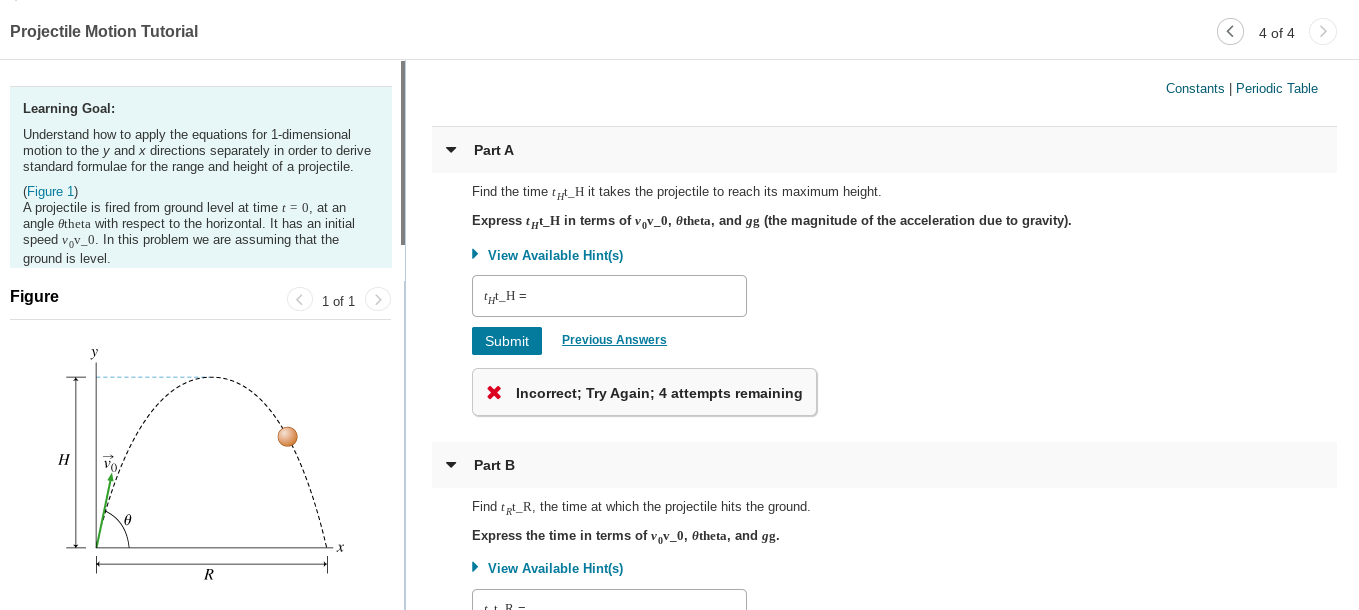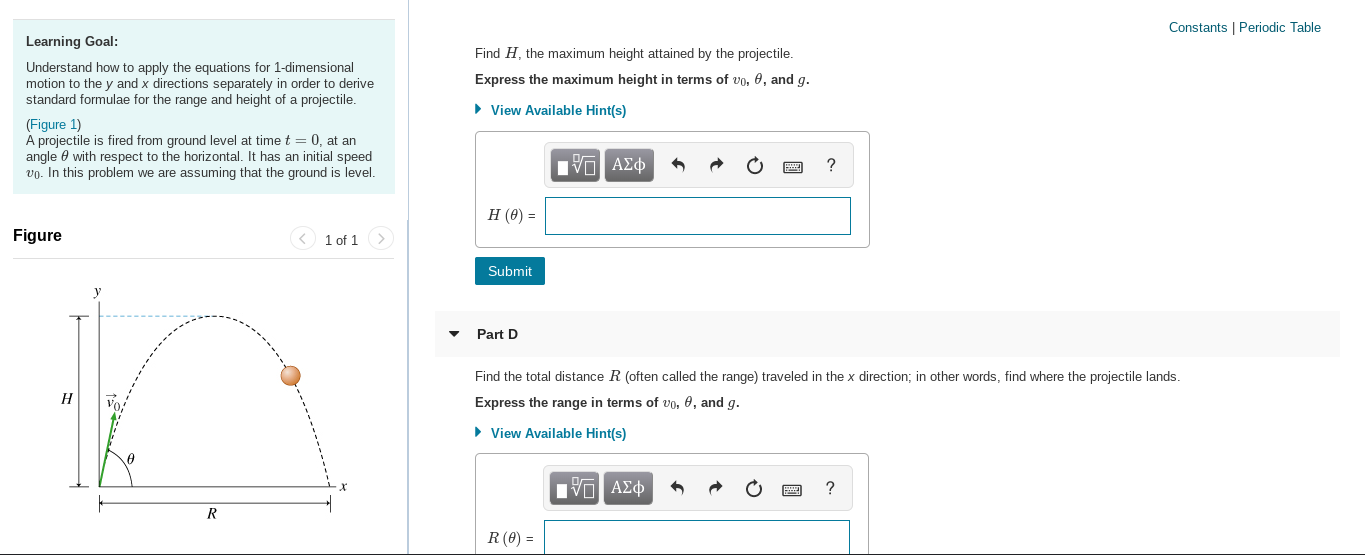# Projectile Motion Tutorial4 of 4Constants | Periodic TableLearning Goal:Understand how to apply the equations for 1-dimensionalmotion to the y and x directions separately in order to derivestandard formulae for the range and height of a projectile.Part A(Figure 1)A projectile is fired from ground level at time t = 0, at anangle Otheta with respect to the horizontal. It has an initialspeed vov_0. In this problem we are assuming that theground is level.Find the time t„t_H it takes the projectile to reach its maximum height.Express ty_H in terms of vov_0, Otheta, and gg (the magnitude of the acceleration due to gravity).• View Available Hint(s)Figure< 1 of 1>'_H =Previous AnswersSubmitX Incorrect; Try Again; 4 attempts remainingНPart BFind tpt_R, the time at which the projectile hits the ground.Express the time in terms of vov_0, Otheta, and gg.• View Available Hint(s) Constants | Periodic TableLearning Goal:Find H, the maximum height attained by the projectile.Understand how to apply the equations for 1-dimensionalmotion to the y and x directions separately in order to derivestandard formulae for the range and height of a projectile.Express the maximum height in terms of vo, 0, and g.• View Available Hint(s)(Figure 1)A projectile is fired from ground level at time t = 0, at anangle e with respect to the horizontal. It has an initial speedvo. In this problem we are assuming that the ground is level.Πν ΑΣφН (0) -< 1 of 1FigureSubmitPart DFind the total distance R (often called the range) traveled in the x direction; in other words, find where the projectile lands.НExpress the range in terms of vo, 0, and g.• View Available Hint(s)ΠV ΑΣφR(0) =

Question
8 views

Hello, can you help me with all the parts in this question, there are 4 total parts attached as 2 screenshots, part A and B is in 1 screenshot and C and D are in the other screenshot. Please make it clear what the answer is for each part.help_outlineImage TranscriptioncloseProjectile Motion Tutorial 4 of 4 Constants | Periodic Table Learning Goal: Understand how to apply the equations for 1-dimensional motion to the y and x directions separately in order to derive standard formulae for the range and height of a projectile. Part A (Figure 1) A projectile is fired from ground level at time t = 0, at an angle Otheta with respect to the horizontal. It has an initial speed vov_0. In this problem we are assuming that the ground is level. Find the time t„t_H it takes the projectile to reach its maximum height. Express ty_H in terms of vov_0, Otheta, and gg (the magnitude of the acceleration due to gravity). • View Available Hint(s) Figure < 1 of 1> '_H = Previous Answers Submit X Incorrect; Try Again; 4 attempts remaining Н Part B Find tpt_R, the time at which the projectile hits the ground. Express the time in terms of vov_0, Otheta, and gg. • View Available Hint(s) fullscreenhelp_outlineImage TranscriptioncloseConstants | Periodic Table Learning Goal: Find H, the maximum height attained by the projectile. Understand how to apply the equations for 1-dimensional motion to the y and x directions separately in order to derive standard formulae for the range and height of a projectile. Express the maximum height in terms of vo, 0, and g. • View Available Hint(s) (Figure 1) A projectile is fired from ground level at time t = 0, at an angle e with respect to the horizontal. It has an initial speed vo. In this problem we are assuming that the ground is level. Πν ΑΣφ Н (0) - < 1 of 1 Figure Submit Part D Find the total distance R (often called the range) traveled in the x direction; in other words, find where the projectile lands. Н Express the range in terms of vo, 0, and g. • View Available Hint(s) ΠV ΑΣφ R(0) = fullscreen
check_circle

Step 1

a)

The required time to reach maximum height is,

Step 2

b)

The required time to reac...

### Want to see the full answer?

See Solution

#### Want to see this answer and more?

Solutions are written by subject experts who are available 24/7. Questions are typically answered within 1 hour.*

See Solution
*Response times may vary by subject and question.
Tagged in

### Mechanical Properties of Matter